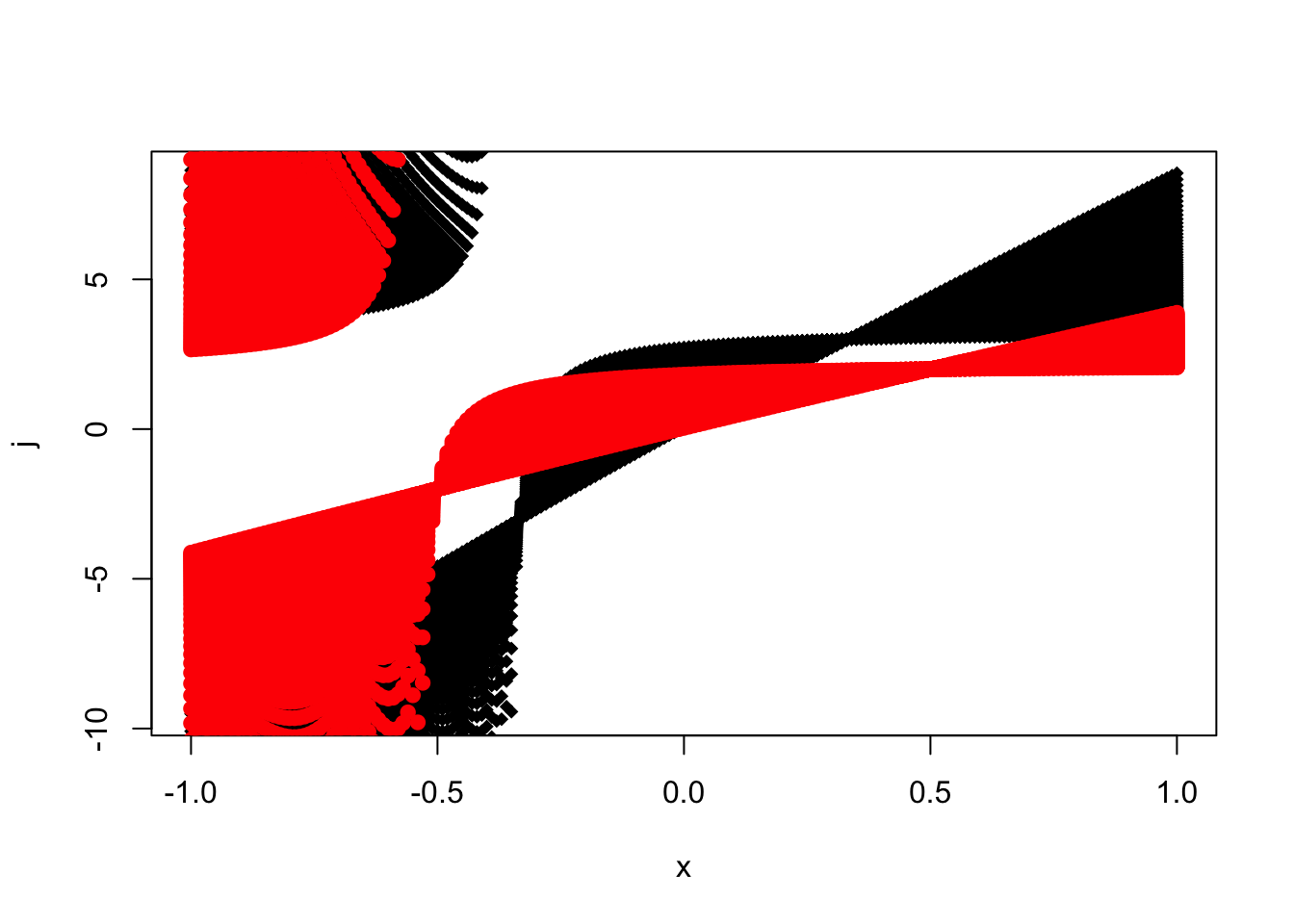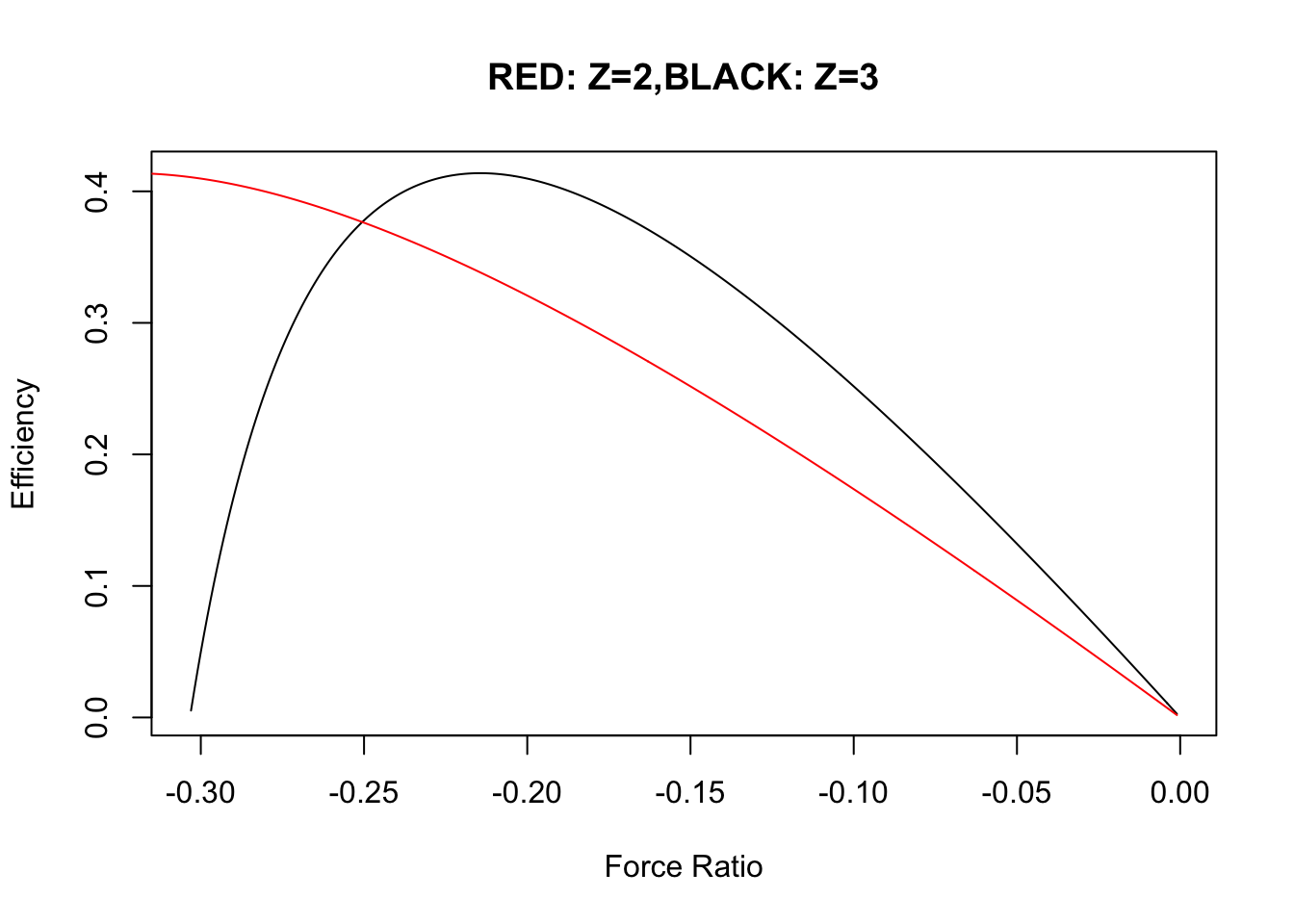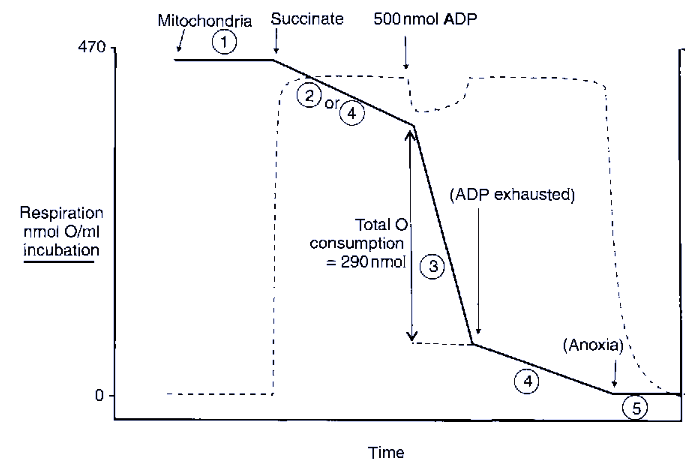## A general output input system

Following the previous arguments in which redox driving force and phosphorylation were respectively the input and output we can express the flux force equation as

\begin{align} J_{i}& = L_i\cdot X_i+ L_{io}\cdot X_o\\ J_{o}&= L_{io}\cdot X_i +L_o \cdot X_o\\ \end{align} ## More about degree and efficiency of coupling

1. \begin{align} \eta&= - \frac{J_o X_o}{J_iX_i }\ \\ &\le 1 \ \end{align}

2. \begin{align} q&= \sqrt{\frac{L_{io}^2}{L_iL_o}} \le 1 \end{align}

## The origin of the inequalities obeyed by degree of coupling and efficiency of coupling

The matrix form of second law is given by

1. $\begin{equation} T\frac{d_iS}{dt}=X^TLX\ge 0 \end{equation}$
Equation clearly indicates that L is a positive definite matrix. This implies, \begin{align} det |L|\ge 0\\ or,\\ L_iL_o-L_{io}^2\ge 0\\ i.e.,\\ q^2 \le 1\\ i.e.,\\ q \le 1 \end{align}

and also, $L_o>0,L_i>0$ as diagonal elements of L matrix is positive.

## Flux Ratio versus the Force Ratio

Let us write the flux ratio and force ratio as $j= \frac{J_{O}}{J_{i}}\\ x= \frac{X_{O}}{X_{i}}\\$ The Stoichimetric coefficient Zis defined as, \begin{align} Z = \sqrt{\frac{L_{o}}{L_{i}}}\\ \end{align}

Given this definition of Stoichiometry , we can write the flux ratios,
(@flowrat) \begin{align} j=\frac{J_o}{J_i}\\ or,\\ j=Z \frac{q +Z(X_o/X_i)}{1 + qZ\frac{X_o}{X_i}}\\ =Z \frac{q +Zx}{1 + qZx}\\ \end{align}

We can express , $j=\frac{L_{o} X_o + L_{io}X_i}{L_{io}X_o + L_{i}X_i}\\ =\frac{Z^2L_iX_o+qzL_iX_i}{L_iqZX_o+L_iX_i}\\=Z.\frac{Zx+q}{qZx+1}$

## Static Head and Level Flow

We can define two classes of steady states.
* Level Flow (free energy of phophorylation is 0) * Static head (e.g. ATP production rate is 0).

Thus we have the following equations. For level flow:
$(J_o/J_i)_{X_o=o} =Zq =L_{io}/L_i\\$ For static head, $(X_o/X_i)_{J_o=0}=-q/Z =-L_{io}/L_o$

We can now see the dependence of flow ratio against flow ratio.\

Z<-3.0

q <- 0.01
for (i in 1:90)
{
q<- q+0.01

x<-seq(-1,1,0.01)
j<- Z*(q+Z*x)/(1+q*Z*x)
if (i==1)
{
plot(x,j,pch=18)
}
else
{
points(x,j,pch=18)
}
}

Z<-2.0

q <- 0.01
for (i in 1:90)
{
q<- q+0.01

x<-seq(-1,1,0.01)
j<- Z*(q+Z*x)/(1+q*Z*x)

points(x,j,pch=19,col="red")

}## Efficiency as a function of Force Ratio

$\eta = - \frac{q+Zx}{q+\frac{1}{Zx}}$

$\eta = - \frac{q-\frac{j}{Z}}{q-\frac{Z}{j}}$

Z<-3.0

q <- 0.01
for (i in 1:90)
{
q<- q+0.01

x<- seq(-1,1,0.001)
j<- Z*(q+Z*x)/(1+q*Z*x)
eta<- -j*x

}
ik<-which(eta>0 & eta<1)

plot(x[ik],eta[ik],type="l",xlab="Force Ratio",ylab="Efficiency",main="RED: Z=2,BLACK: Z=3")
Z<-2.0

q <- 0.01
for (i in 1:90)
{
q<- q+0.01

x<- seq(-1,1,0.001)
j<- Z*(q+Z*x)/(1+q*Z*x)
eta<- -j*x

}
ik<-which(eta>0 & eta<1)

points(x[ik],eta[ik],type="l",col="red")It may be interesting to see that there is an optimal force ratio at which the efficiency is maximal . Secondly in a wide spectrum of force ratio staring from -3 to +3 , the efficiecincy seems to be meaningful within a force ratio (x) range The efficiency is chosen in the range $$\eta \le 0.3$$ for Z=2,3.All the other values of efficiency are ruled out by the second law as they would not satisfy the $$\eta \le 1$$.

## Problem

Find the value of x at Z=jWhen oxygen electrode was first being applied to mitochondrian studies the following conditions were fulfilled:
* State 1 : mitochondria alone
* State 2 : Substrate added , respiration low owing to lack of ADP.
* State 3 : A limited amount of ADP added allowing rapid respiration
* State 4 All ADP converted to ATP ,respiration shows
* State 5: Anoxia

## Problem

Describe the flux flow status of the above.